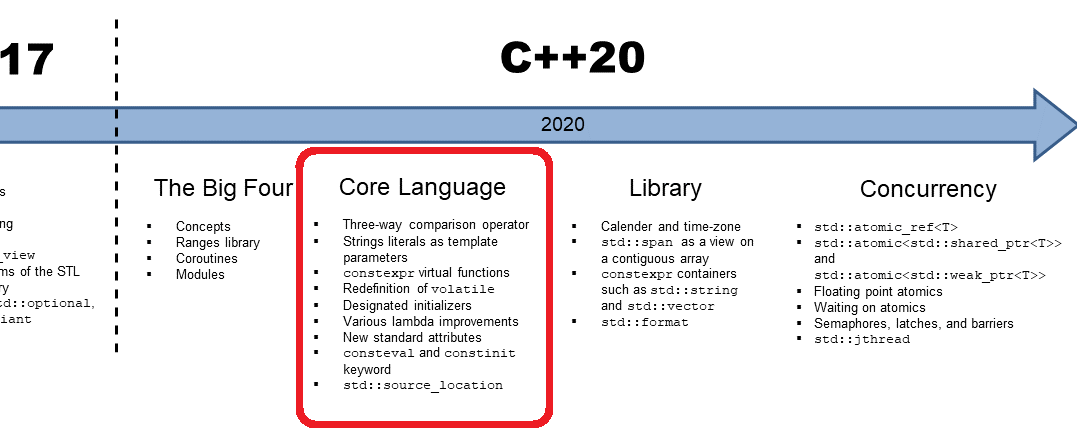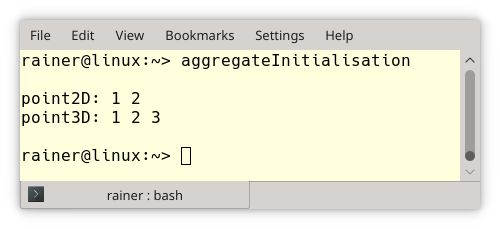# C++ 20: The Core Language

My last post C++20: The Big Four started with an overview of concepts, ranges, coroutines, and modules. Of course, C++20 has more to offer. Today, let’s continue my overview of the core language.## Core Language

Looking at the image, you see the features I want to cover.

### The three-way Comparison operator <=>

The three-way comparison operator <=> is often just called the spaceship operator. The spaceship operator determines whether A < B, A = B, or A > B for two values A and B.

The compiler can auto-generate the three-way comparison operator. You have only to ask for it politely with default. In this case, you get all six comparison operators such as ==, !=, <, <=, >, and >=.

```#include <compare>
struct MyInt {
int value;
MyInt(int value): value{value} { }
auto operator<=>(const MyInt&) const = default;
};
```

The default operator <=> performs lexicographical comparison starting the base classes left to right and using the non-static members in declaration order. Here is a quite sophisticated example from the Microsoft blog: Simplify Your Code with Rocket Science: C++ 20’s Spaceship Operator.

##Modernes C++ Mentoring

Be part of my mentoring programs:

• "Fundamentals for C++ Professionals" (open)
• "Design Patterns and Architectural Patterns with C++" (open)
• "C++20: Get the Details" (reopens December 2023)
• Do you want to stay informed about my mentoring programs: Subscribe via E-Mail.

```struct Basics {
int i;
char c;
float f;
double d;
auto operator<=>(const Basics&) const = default;
};

struct Arrays {
int ai;
char ac;
float af;
auto operator<=>(const Arrays&) const = default;
};

struct Bases : Basics, Arrays {
auto operator<=>(const Bases&) const = default;
};

int main() {
constexpr Bases a = { { 0, 'c', 1.f, 1. },
{ { 1 }, { 'a', 'b' }, { 1.f, 2.f, 3.f }, { { 1., 2. }, { 3., 4. } } } };
constexpr Bases b = { { 0, 'c', 1.f, 1. },
{ { 1 }, { 'a', 'b' }, { 1.f, 2.f, 3.f }, { { 1., 2. }, { 3., 4. } } } };
static_assert(a == b);
static_assert(!(a != b));
static_assert(!(a < b));
static_assert(a <= b);
static_assert(!(a > b));
static_assert(a >= b);
}
```

I assume the most complicated stuff in this code snippet is not the spaceship operator but the initialization of Base using aggregate initialization. Aggregate initialization essential means that you can directly initialize the members of class types (class, struct, or union) if all members are public. You can use, in this case, a braced-initialization list such as in the example. Okay, this was a simplification. Read the details here: aggregate initialization

### String Literals as Template Parameters

Before C++20, you could not use a string as a non-type template parameter. With C++20, you can use it. The idea is to use the standard defined basic_fixed_string, which has a constexpr constructor. The constexpr constructor enables it to instantiate the fixed string at compile-time.

```template<std::basic_fixed_string T>
class Foo {
static constexpr char const* Name = T;
public:
void hello() const;
};

int main() {
Foo<"Hello!"> foo;
foo.hello();
}
```

### constexpr Virtual Functions

Virtual functions can not be invoked in constant expressions because the dynamic type is unknown. This restriction will fall with C++20.

### Designated initializers

Let me first write about aggregate initialization. Here is a straightforward example.

```// aggregateInitialisation.cpp

#include <iostream>

struct Point2D{
int x;
int y;
};

class Point3D{
public:
int x;
int y;
int z;
};

int main(){

std::cout << std::endl;

Point2D point2D {1, 2};
Point3D point3D {1, 2, 3};

std::cout << "point2D: " << point2D.x << " " << point2D.y << std::endl;
std::cout << "point3D: " << point3D.x << " " << point3D.y << " " << point3D.z << std::endl;

std::cout << std::endl;

}
```

I assume an explanation of the program is not necessary. Here is the output of the program:Explicit is better than implicit. Let’s see what that means. The initialization in the program aggregateInitialisation.cpp is quite error-prone because you can swap the constructor arguments, and you will never notice. Here designated initializers from C99 kick in.

```// designatedInitializer.cpp

#include <iostream>

struct Point2D{
int x;
int y;
};

class Point3D{
public:
int x;
int y;
int z;
};

int main(){

std::cout << std::endl;

Point2D point2D {.x = 1, .y = 2};
// Point2D point2d {.y = 2, .x = 1};         // (1) error
Point3D point3D {.x = 1, .y = 2, .z = 2};
// Point3D point3D {.x = 1, .z = 2}          // (2)  {1, 0, 2}

std::cout << "point2D: " << point2D.x << " " << point2D.y << std::endl;
std::cout << "point3D: " << point3D.x << " " << point3D.y << " " << point3D.z << std::endl;

std::cout << std::endl;

}
```

The arguments for the instances of Point2d and Point3D are explicitly named. The program’s output is identical to the output of the program aggregateInitialisation.cpp. The commented outlines (1) and (2) are pretty interesting. Line (1) would give an error because the order of the designator does not match their declaration order. The designator for y is missing inline (3). In this case, y would be initialized to 0 using braces-initialization-list {1, 0, 3}.

### Various Lambda Improvements

Lambas will have many improvements in C++20.

If you want more details, go to Bartek’s post about lambda improvements in C++17 and C++20 or wait for my detailed posts. Anyway, here are two exciting changes we will get.

• Allow [=, this] as lambda capture and deprecate implicit capture of this via [=]

```struct Lambda {
auto foo() {
return [=] { std::cout << s << std::endl; };
}

std::string s;
};

struct LambdaCpp20 {
auto foo() {
return [=, this] { std::cout << s << std::endl; };
}

std::string s;
};
```

The implicit [=] capture by copy inside the struct Lambda causes in C++20 a deprecation warning. You don’t get with C++20 a deprecation warning when you capture this explicitly by copying [=, this].

• Template lambdas

Your first impression, like mine may be: Why do we need template lambdas? When you write a generic lambda with C++14 [](auto x){ return x; }, the compiler automatically generates a class with a templatized call operator:

```template <typename T>
T operator(T x) const {
return x;
}
```

Sometimes, you want to define a lambda that works only for a specific type, such as a std::vector. Now, template lambdas have come to our rescue. Instead of a type parameter, you can also use a concept:

```auto foo = []<typename T>(std::vector<T> const& vec) {
// do vector specific stuff
};
```

### New Attributes: [[likely]] and [[unlikely]]

With C++20, we get new attributes [[likely]] and [[unlikely]]. Both attributes allow it to give the optimizer a hint of whether the path of execution is more or less likely.

```for(size_t i=0; i < v.size(); ++i){
if (v[i] < 0) [[likely]] sum -= sqrt(-v[i]);
else sum += sqrt(v[i]);
}
```

### consteval and constinit Specifier

The new specifier consteval creates an immediate function. For an immediate function, every call to the function must produce a compile-time constant expression. An immediate function is implicit a constexpr function.

```consteval int sqr(int n) {
return n*n;
}
constexpr int r = sqr(100);  // OK

int x = 100;
int r2 = sqr(x);             // Error
```

The final assignment gives an error because x is not a constant expression and, therefore, sqr(x) can not  be performed at compile-time

constinit ensures that the variable with static storage duration is initialized at compile-time. Static storage duration means that the object is allocated when the program begins and deallocated when the program ends. Objects declared at namespace scope (global objects), declared as static or extern have static storage duration.

### std::source_location

C++11 has two macros for __LINE__ and __FILE__ to get the information when the macros are used. With C++20, the class source_location gives you the file name, the line number, the column number, and the function name of the source code. The short example from cppreference.com shows the first usage:

```#include <iostream>
#include <string_view>
#include <source_location>

void log(std::string_view message,
const std::source_location& location = std::source_location::current())
{
std::cout << "info:"
<< location.file_name() << ":"
<< location.line() << " "
<< message << '\n';
}

int main()
{
log("Hello world!");  // info:main.cpp:15 Hello world!
}
```

## What’s next?

This post was the first overview of the more minor features of the core language. The next post continues my story with the library features in C++20.

Thanks a lot to my Patreon Supporters: Matt Braun, Roman Postanciuc, Tobias Zindl, G Prvulovic, Reinhold Dröge, Abernitzke, Frank Grimm, Sakib, Broeserl, António Pina, Sergey Agafyin, Андрей Бурмистров, Jake, GS, Lawton Shoemake, Jozo Leko, John Breland, Venkat Nandam, Jose Francisco, Douglas Tinkham, Kuchlong Kuchlong, Robert Blanch, Truels Wissneth, Kris Kafka, Mario Luoni, Friedrich Huber, lennonli, Pramod Tikare Muralidhara, Peter Ware, Daniel Hufschläger, Alessandro Pezzato, Bob Perry, Satish Vangipuram, Andi Ireland, Richard Ohnemus, Michael Dunsky, Leo Goodstadt, John Wiederhirn, Yacob Cohen-Arazi, Florian Tischler, Robin Furness, Michael Young, Holger Detering, Bernd Mühlhaus, Matthieu Bolt, Stephen Kelley, Kyle Dean, Tusar Palauri, Dmitry Farberov, Juan Dent, George Liao, Daniel Ceperley, Jon T Hess, Stephen Totten, Wolfgang Fütterer, Matthias Grün, Phillip Diekmann, Ben Atakora, Ann Shatoff, Rob North, and Bhavith C Achar.

Thanks, in particular, to Jon Hess, Lakshman, Christian Wittenhorst, Sherhy Pyton, Dendi Suhubdy, Sudhakar Belagurusamy, Richard Sargeant, Rusty Fleming, John Nebel, Mipko, Alicja Kaminska, Slavko Radman, and David Poole.

 My special thanks to EmbarcaderoMy special thanks to PVS-StudioMy special thanks to Tipi.buildMy special thanks to Take Up Code## Seminars

I’m happy to give online seminars or face-to-face seminars worldwide. Please call me if you have any questions.

### Standard Seminars (English/German)

Here is a compilation of my standard seminars. These seminars are only meant to give you a first orientation.

• C++ – The Core Language
• C++ – The Standard Library
• C++ – Compact
• C++11 and C++14
• Concurrency with Modern C++
• Design Pattern and Architectural Pattern with C++
• Embedded Programming with Modern C++
• Generic Programming (Templates) with C++

#### New

• Clean Code with Modern C++
• C++20

### Contact Me

Modernes C++ Mentoring,Tags:
0 replies